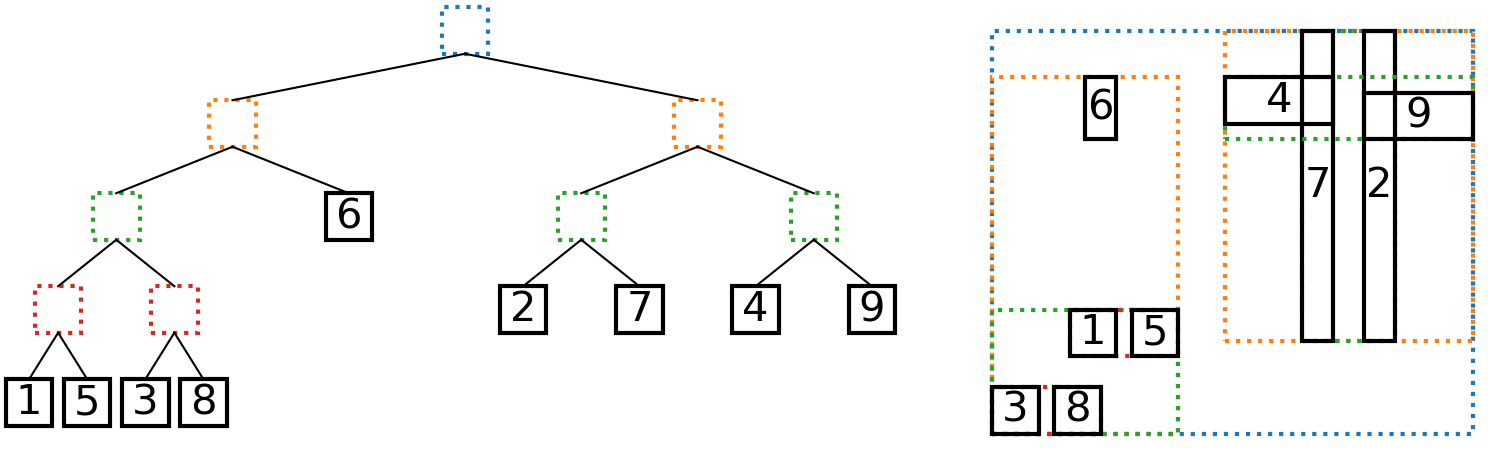AABBTree - Axis-Aligned Bounding Box Trees¶

AABBTree is a pure Python implementation of a static d-dimensional axis aligned bounding box (AABB) tree. It is inspired by Introductory Guide to AABB Tree Collision Detection from Azure From The Trenches.Left: An AABB tree, leaves numbered by insertion order. Right: The AABBs and their bounding boxes.

Installation¶

AABBTree is available through PyPI and can be installed by running:

pip install aabbtree


To test that the package installed properly, run:

python -c "import aabbtree"


Alternatively, the package can be installed from source by downloading the latest release from the AABBTree repository on GitHub. Extract the source and, from the top-level directory, run:

pip install -e .


The --user flag may be needed, depending on permissions.

Example¶

The following example shows how to build an AABB tree and test for overlap:

>>> from aabbtree import AABB
>>> from aabbtree import AABBTree
>>> tree = AABBTree()
>>> aabb1 = AABB([(0, 0), (0, 0)])
>>> aabb2 = AABB([(-1, 1), (-1, 1)])
>>> aabb3 = AABB([(4, 5), (2, 3)])
>>> tree.does_overlap(aabb2)
True
>>> tree.overlap_values(aabb2)
['box 1']
>>> tree.does_overlap(aabb3)
False
>>> print(tree)
AABB: [(0, 5), (0, 3)]
Value: None
Left:
AABB: [(0, 0), (0, 0)]
Value: box 1
Left: None
Right: None
Right:
AABB: [(4, 5), (2, 3)]
Value: None
Left: None
Right: None


API¶

class aabbtree.AABB(limits=None)[source]

Bases: object

Axis-aligned bounding box (AABB)

The AABB is a d-dimensional box.

Parameters: limits (iterable, optional) – The limits of the box. These should be specified in the following manner: limits = [(xmin, xmax), (ymin, ymax), (zmin, zmax), ...]  The default value is None.
classmethod merge(aabb1, aabb2)[source]

Merge AABB

Find the AABB of the union of AABBs.

Parameters: aabb1 (AABB) – An AABB aabb2 (AABB) – An AABB An AABB that contains both of the inputs AABB
overlap_volume(aabb)[source]

Determine volume of overlap between AABBs

Let $$\left(l_i^{(1)}, u_i^{(1)}\right)$$ be the i-th dimension lower and upper bounds for AABB 1, and let $$\left(l_i^{(2)}, u_i^{(2)}\right)$$ be the lower and upper bounds for AABB 2. The volume of overlap is:

$V = \prod_{i=1}^n \text{max}\left(0, \text{min}\left(u_i^{(1)}, u_i^{(2)}\right) - \text{max}\left(l_i^{(1)}, l_i^{(2)}\right) \right)$
Parameters: aabb (AABB) – The AABB to calculate for overlap volume Volume of overlap float
overlaps(aabb)[source]

Determine if two AABBs overlap

Parameters: aabb (AABB) – The AABB to check for overlap Flag set to true if the two AABBs overlap bool
perimeter

float – perimeter of AABB

The perimeter $$p_n$$ of an AABB with side lengths $$l_1 \ldots l_n$$ is:

$\begin{split}p_1 &= 0 \\ p_2 &= 2 (l_1 + l_2) \\ p_3 &= 2 (l_1 l_2 + l_2 l_3 + l_1 l_3) \\ p_n &= 2 \sum_{i=1}^n \prod_{j=1\neq i}^n l_j\end{split}$
volume

float – volume of AABB

The volume $$V_n$$ of an AABB with side lengths $$l_1 \ldots l_n$$ is:

$\begin{split}V_1 &= l_1 \\ V_2 &= l_1 l_2 \\ V_3 &= l_1 l_2 l_3 \\ V_n &= \prod_{i=1}^n l_i\end{split}$
class aabbtree.AABBTree(aabb=AABB(None), value=None, left=None, right=None)[source]

Bases: object

Static AABB Tree

An AABB tree where the bounds of each AABB do not change.

Parameters: aabb (AABB) – An AABB value – The value associated with the AABB left (AABBTree, optional) – The left branch of the tree right (AABBTree, optional) – The right branch of the tree
add(aabb, value=None, method='volume')[source]

This function inserts a node into the AABB tree. The function chooses one of three options for adding the node to the tree:

• Add it to the left side
• Add it to the right side
• Become a leaf node

The cost of each option is calculated based on the method keyword, and the option with the lowest cost is chosen.

Parameters: aabb (AABB) – The AABB to add. value – The value associated with the AABB. Defaults to None. method (str) – The method for deciding how to build the tree. Should be one of the following: volume volume Costs based on total bounding volume and overlap volume Let $$p$$ denote the parent, $$l$$ denote the left child, $$r$$ denote the right child, $$x$$ denote the AABB to add, and $$V$$ be the volume of an AABB. The three options to add $$x$$ to the left branch, add it to the right branch, or create a new parent. The cost associated with each of these options is: $\begin{split}C(\text{add left}) &= V(p \cup x) - V(p) + V(l \cup x) - V(l) + V((l \cup x) \cap r) \\ C(\text{add right}) &= V(p \cup x) - V(p) + V(r \cup x) - V(r) + V((r \cup x) \cap l) \\ C(\text{create parent}) &= V(p \cup x) + V(p \cap x)\end{split}$ In the add-left cost, the term $$V(b \cup x) - V(b)$$ is the increase in parent bounding volume. The cost $$V(l \cup x) - V(l)$$ is the increase in left child bounding volume. The last term, $$V((l \cup x) \cap r)$$ is the overlapping volume between children if $$x$$ were added to the left child. The cost to create a new parent is the bounding volume of the parent and $$x$$ plus their overlap volume. This cost function includes the increases in bounding volumes and the amount of overlap- two values a balanced AABB tree should minimize. The cost function suits the author’s current needs, though other applications may seek different tree properties. Please visit the AABBTree repository if interested in implementing another cost function.
does_overlap(aabb)[source]

Check for overlap

This function checks if the limits overlap any leaf nodes in the tree. It returns true if there is an overlap.

Parameters: aabb (AABB) – The AABB to check. True if overlaps with a leaf node of tree. bool
overlap_values(aabb)[source]

Get values of overlapping AABBs

This function gets the value field of each overlapping AABB.

Parameters: aabb (AABB) – The AABB to check. Value fields of each node that overlaps. list
depth

int – Depth of the tree

is_leaf

bool – returns True if is leaf node

Contributing¶

Contributions to the project are welcome. Please visit the AABBTree repository to clone the source files, create a pull request, and submit issues.